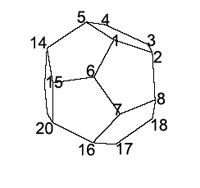In my geometry 101 course I'm doing the rotation-symmetry groups
of the Platonic solids right now. This goes slightly slower than
expected as it turned out that some secondary schools no longer give a
formal definition of what a group is. So, a lot of time is taken up
explaining permutations and their properties as I want to view the
Platonic groups as subgroups of the permutation groups on the vertices.
To prove that the _tetrahedral group_ is isomorphic to $A_4$ was pretty
straigthforward and I'm half way through proving that the
_octahedral group_ is just $S_4$ (using the duality of the octahedron
with the cube and using the $4$ body diagonals of the cube).
Next
week I have to show that the _icosahedral group_ is isomorphic to $A_5$
which is a lot harder. The usual proof (that is, using the duality
between the icosahedron and the dodecahedron and using the $5$ cubes
contained in the dodecahedron, one for each of the diagonals of a face)
involves too much calculations to do in one hour. An alternative road is
to view the icosahedral group as a subgroup of $S_6$ (using the main
diagonals on the $12$ vertices of the icosahedron) and identifying this
subgroup as $A_5$. A neat exposition of this approach is given by John Baez in his
post Some thoughts on
the number $6$
. (He also has another post on the icosahedral group
in his Week 79's
finds in mathematical physics
).But
probably I'll go for an “In Gap we
thrust”-argument. Using the numbers on the left, the rotation by
$72^o$ counter-clockwise in the top face we get the permutation in
$S_{20}$
$(1,2,3,4,5)(6,8,10,12,14)(7,9,11,13,15)(16,17,18,19,20)$
and the
rotation by $72^o$ counterclockwise along the face $(1,2,8,7,8)$ gives
the permutation
$(1,6,7,8,2)(3,5,15,16,9)(4,14,20,17,10)(12,13,19,18,11)$
GAP
calculates that the subgroup $dode$ of $S_{20}$ generated by these two
elements is $60$ (the correct number) and with $IsSimplegroup(dode);$ we
find that this group must be simple. Finally using
$IsomorphismTypeInfoFiniteSimplegroup(dode);$
we get the required
result that the group is indeed isomorphic to $A_5$. The time saved I
can then use to tell something about the classification project of
finite simple groups which might be more inspiring than tedious
calculations…

### Similar Posts:

Published in featured